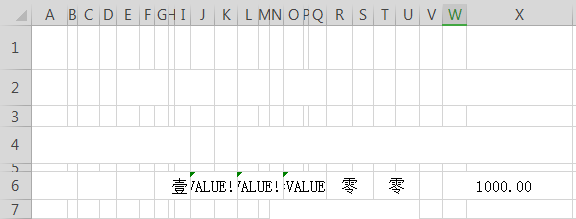# 求教大神！为何下面公式遇到0就无法显示大写零出来的?显示Value！谢谢J6=IF(IF(LEN(INT(W6))>=3,LEFT(RIGHT(INT(W6),3)),"")="","",CHOOSE(IF(IF(LEN(INT(W6))>=3,LEFT(RIGHT(INT(W6),3)),"")=0,10,IF(LEN(INT(W6))>=3,LEFT(RIGHT(INT(W6),3)),"")),"壹","贰","叁","肆","伍","陆","柒","捌","玖","零"))

L6=IF(IF(LEN(INT(W6))>=2,LEFT(RIGHT(INT(W6),2)),"")="","",CHOOSE(IF(IF(LEN(INT(W6))>=2,LEFT(RIGHT(INT(W6),2)),"")=0,10,IF(LEN(INT(W6))>=2,LEFT(RIGHT(INT(W6),2)),"")),"壹","贰","叁","肆","伍","陆","柒","捌","玖","零"))

O6=IF(IF(LEN(INT(W6))>=1,IF(INT(W6)=0,"",RIGHT(INT(W6))),"")="","",CHOOSE(IF(IF(LEN(INT(W6))>=1,IF(INT(W6)=0,"",RIGHT(INT(W6))),"")=0,11,IF(LEN(INT(W6))>=1,IF(INT(W6)=0,"",RIGHT(INT(W6))),"")),"壹","贰","叁","肆","伍","陆","柒","捌","玖","零"))

，具体解决方案如下：

J6:=IF(IF(W6上百,百位,"")="","",CHOOSE(IF(IF(W6上百,百位,"")=0,10,IF(W6上百,百位,"")),"壹","贰","叁","肆","伍","陆","柒","捌","玖","零"))

=IF(IF(W6不上百,百位,"",CHOOSE(IF(IF(W6上百,百位,"")=0,10,IF(W6上百,百位,"")),"壹","贰","叁","肆","伍","陆","柒","捌","玖","零"))

=IF(IF(LEN(INT(W6))>=3,LEFT(RIGHT(INT(W6),3)),"")="","",CHOOSE(IF(IF(LEN(INT(W6))>=3,LEFT(RIGHT(INT(W6),3)),"")=0,10,IF(LEN(INT(W6))>=3,LEFT(RIGHT(INT(W6),3)),"")),"壹","贰","叁","肆","伍","陆","柒","捌","玖","零"))

（1）

IF(IF(LEN(INT(W6))>=3,LEFT(RIGHT(INT(W6),3)),"")="","",  ……

IF(W6<100,"", ……

（2）

IF(IF(LEN(INT(W6))>=3,LEFT(RIGHT(INT(W6),3)),"")=0,10, ……

IF(IF(LEN(INT(W6))>=3,LEFT(RIGHT(INT(W6),3)),"")="0",10, ……

IF(IF(LEN(INT(W6))>=3,--LEFT(RIGHT(INT(W6),3)),"")=0,10, ……

IF(--LEFT(RIGHT(INT(W6),3))=0,10, ……

=IF(W6<100,"",CHOOSE(LEFT(RIGHT(INT(W6),3))+1,"零","壹","贰","叁","肆","伍","陆","柒","捌","玖"))

=IF(W6<100,"",TEXT(LEFT(RIGHT(INT(W6),3)),"[DBNum2][\$-804]G/通用格式"))

=TEXT(w6,"[dbnum2]")&"元整"

Excle中，使用SUMIFS,明明公式输对了，可是不能显示数值求大神指教。

1、将光标点到B3单元格，鼠标点击公式选项卡。2、点击插入函数。3、点击插入sumifs函数。4、求和范围中点击费用报销管理，鼠标拖动选中G3-G401区域。5、求和条件中点击费用管理-日期即A3-A401区域，限定值中输入>=2013-4-1。6、依次输入求和区域与限定值的值，完成图如下。7、点击确定，可以看到求和公式变为下图。8、返回统计表最终得到下图。1、使用插入函数向导可以只用鼠标选中区域，不用键盘输入，避免中英文符号混用。

2、不要改变向导内已经输入的英文符号。

3、不要选错条件区域。

EXCEL表中 找同名称的最小值和最大值,求教大神们!谢谢

=MAX((\$A\$2:\$A\$10="A")*(\$B\$2:\$B\$10))

=MIN((\$A\$2:\$A\$10="A")*(\$B\$2:\$B\$10))